You are now in the main content area

# Math & Science

## Creating accessible math and science equations

This page discusses tools for creating accessible equations to support a more inclusive learning environment. We recommend the following platforms to create accessible equations and formulas:

• Microsoft Word: Best for assignments and project handouts.
• D2L Brightspace: Best for online learning modules and quizzes.

### Benefits of accessible equations

• Allows screen readers to read equations with proper mathematical meaning.
• Allows editing equations on the platform which includes manipulating, replacing and changing the equation without rewriting it.
• The math is searchable, making it easier for others to find specific equations.
• Math languages such as MathML, UnicodeMath, LaTeX can be used to create accessible equations in these platforms.

### Best practices 

• When creating equations in Microsoft Word, it's recommended to leave it in the original (.docx) format, as opposed to saving as PDF. Learn more below: recommended format.
• For a mathematical solution that requires steps to solve it, insert one equation per line instead of creating one big equation with multiple lines.
• Always use the equation builder to create equations as it provides the best support.
• When not feasible, add alt text to images of equations.
• Try to insert symbols using the equation editor instead of copying one from elsewhere.
• Text copied from other sources may not be fully supported by assistive technologies.## Accessible equations in Microsoft Word

Microsoft Word offers an equation builder that provides good support for assistive technologies. Equations can be created using the equation menu, LaTeX, and UnicodeMath.

Note: The latest version of Microsoft Office 365 is required. Office 365 is available to all students, staff, and faculty.

### Recommended format

It's recommended to save documents in the default Microsoft Word (.docx) format, as it offers the most accessibility for students. Students can adjust the font size, improve colour contrast if needed, and leverage the Read Aloud feature.

PDF format does not offer good assistive technology support for documents containing equations. If you choose to save as PDF, ensure you keep a backup of the original Word document. Alternatively, you can share both formats!

### Writing equations in Microsoft Word

#### Step 1

1. Click the Insert tab on the navigation bar.
2. Click the Symbols button.
3. Select Equations.
4. Click Insert New Equation.

Tip: Use the Keyboard shortcut Alt + = to insert an equation.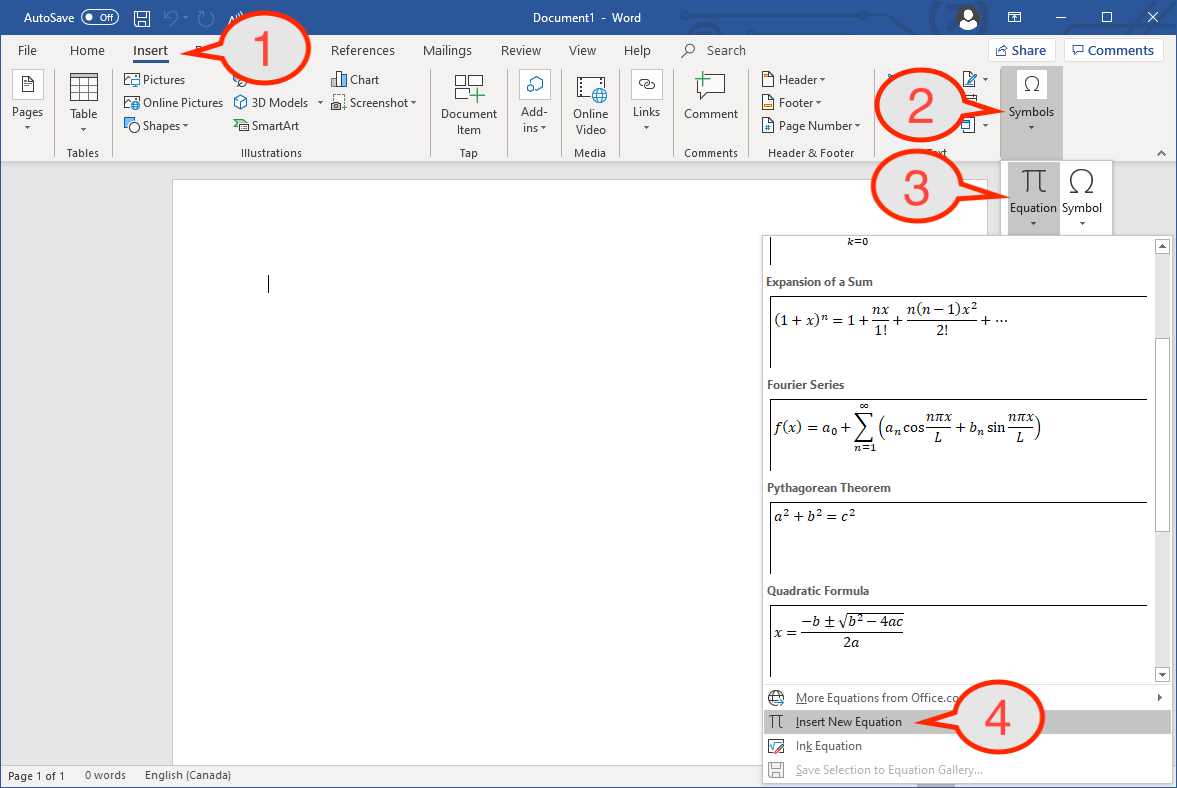#### Step 2

After creating the equation block, navigate to the Design tab to play around with different features for writing equations.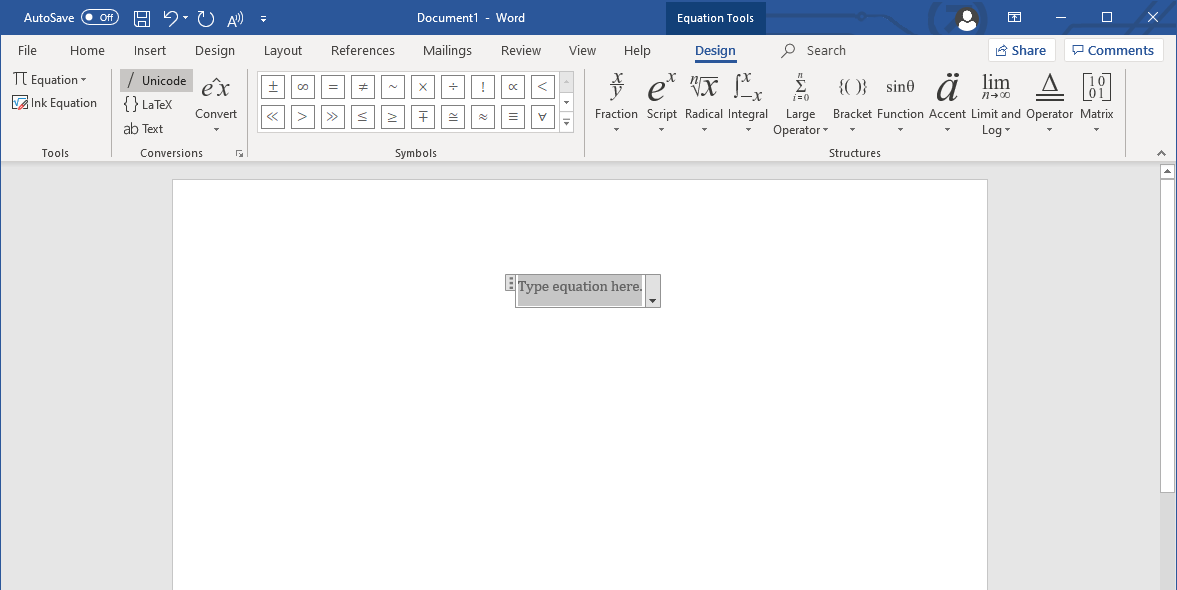## Accessible equations in D2L Brightspace

The D2L Brightspace equation editor uses MathType and MathJax to support an accessible learning environment and offers support for various assistive technologies.

• Creating equations is quick and easy.
• Editing or revising equations is easy using the editor, and will update instantly once published.
• This allows other instructors or teaching assistants to easily edit equations if needed.
• Eliminates the need to re-upload multiple Word documents.
• Students have the option to download these documents as HTML files for offline use.
• Assistive technology can read the equations as structural properties are still available.

### Writing equations in D2L Brightspace

#### Step 1

Create a File in your Brightspace module.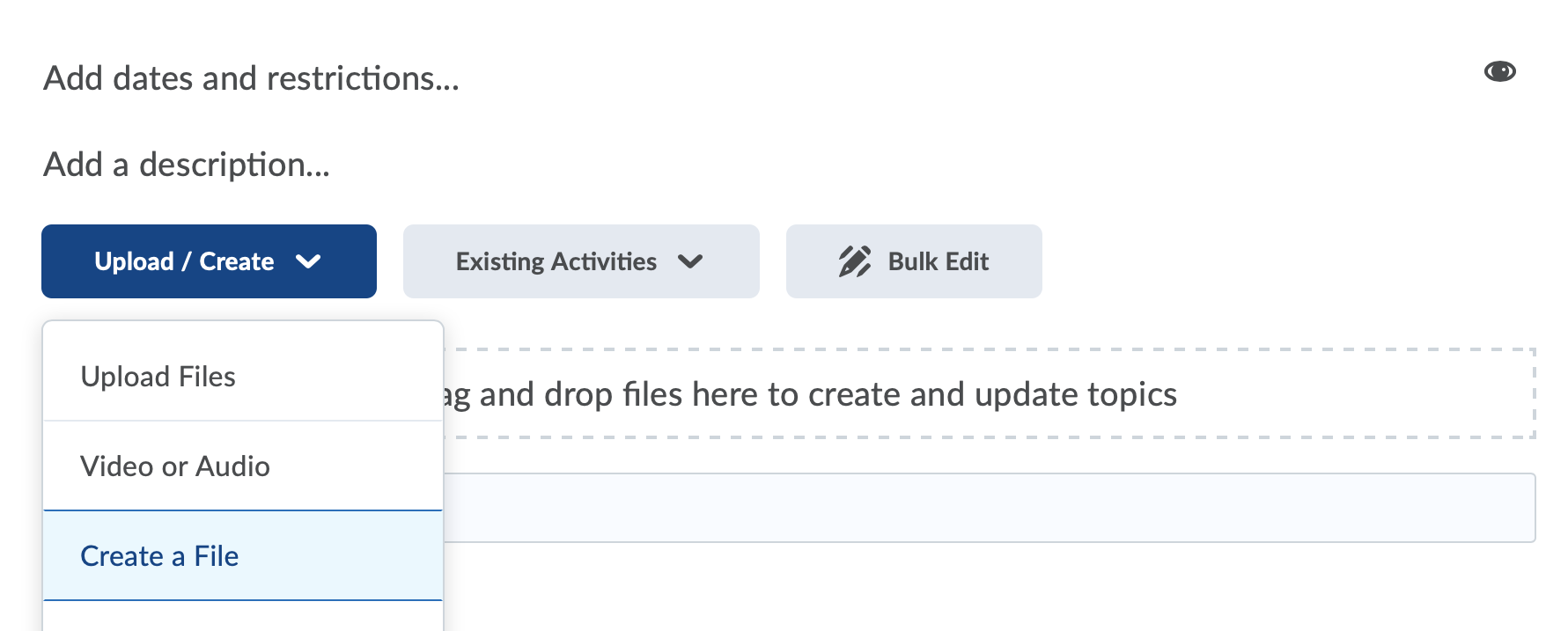#### Step 2

1. Click the Show All Components button (icon with three dots) in the editor menu.
2. Click the Graphical Equation button (Sigma icon) to start writing an equation using the Graphical Equation Editor.
1. Alternatively, you can click More Equation Actions (arrow beside Sigma icon) to select a different equation editor.

Tip: Graphical Equation is the easiest to use but learning how to write LaTeX may be more efficient. They both provide the same level of accessibility in D2L Brightspace.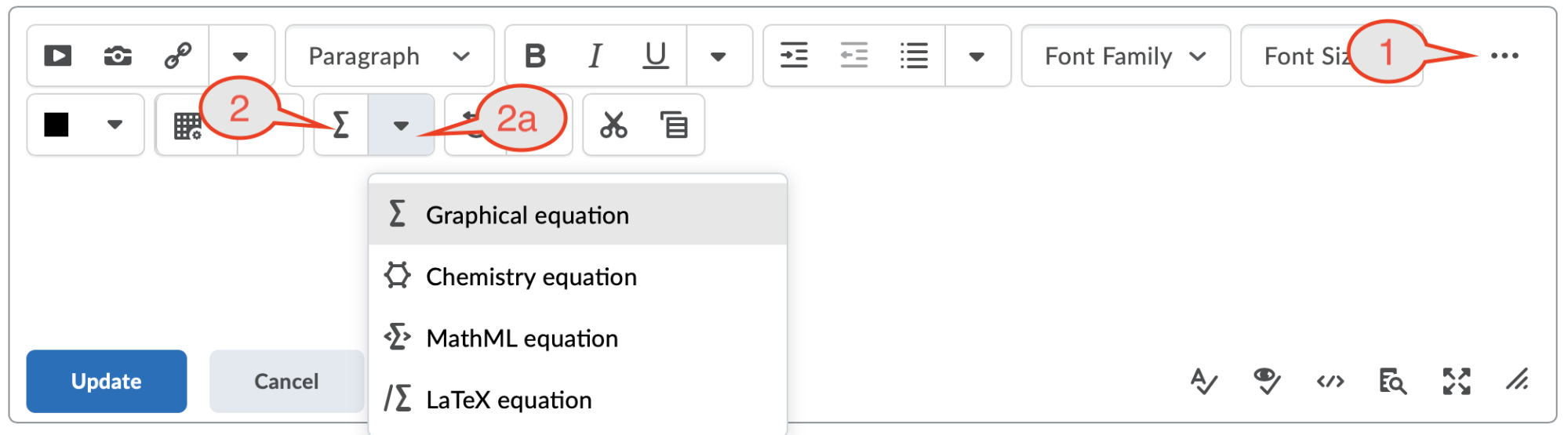#### Step 3

Type the equation you want to insert using the appropriate markup language you selected and click Insert.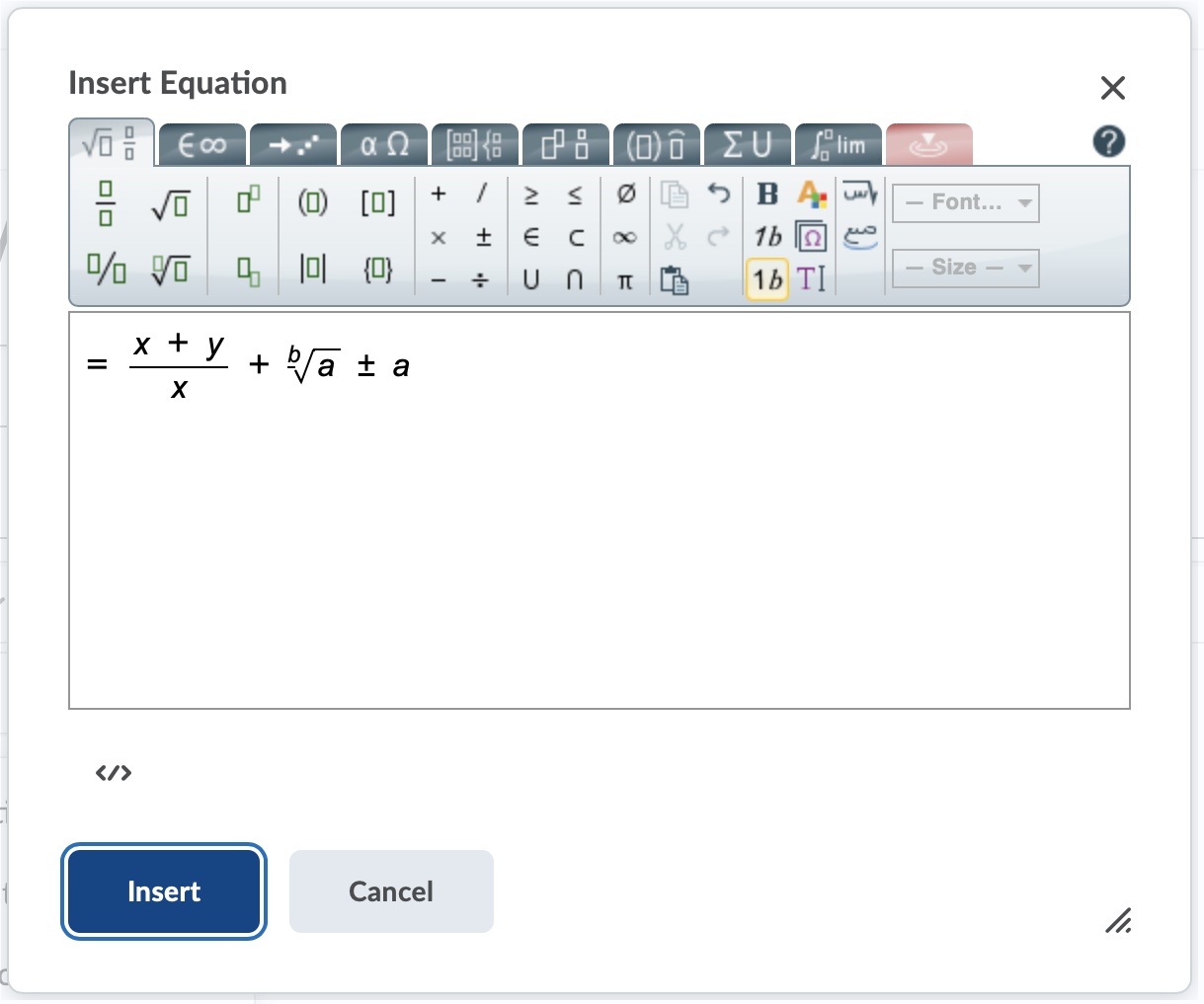### Editing equations in D2L Brightspace

1. Select the equation you want to edit by clicking on the equation or highlighting it using your mouse.
2. Click the Equation button to start editing the equation
1. The equation button referred here is dependent on the type of the equation. It can be a “Graphical Equation”, “Chemical Equation”, “MathML Equation” or “LaTeX Equation” button.
3. Click Insert once you have finished editing the equation.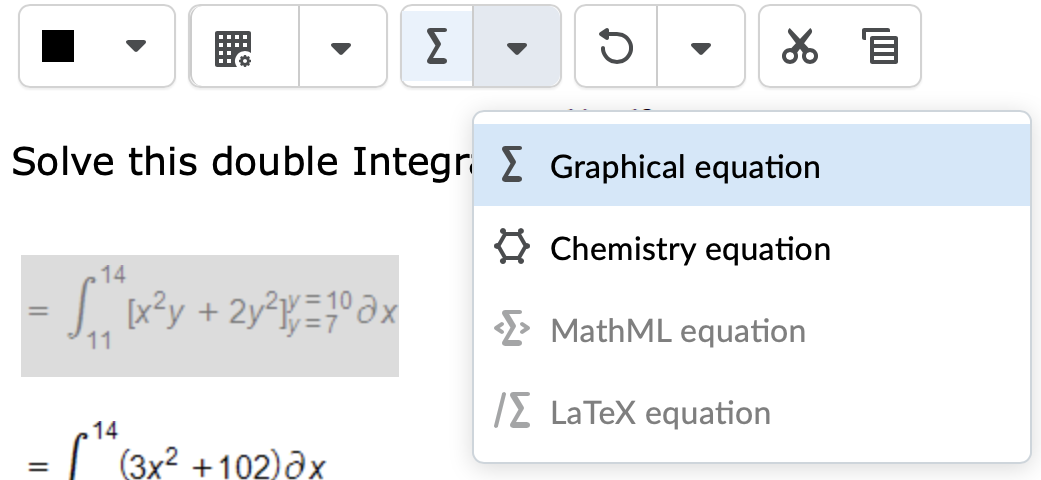For more comprehensive information on MathType, please visit how to use the MathType editor, external link.

### Reading equations in D2L Brightspace using a screen reader

MathML is a developing specification for writing math on the web, which means that most browsers and screen readers will have limitations when reading math elements in D2L Brightspace.

Disclaimer: The following section was prepared June 2020. Experiences may vary with different versions or updates.

#### Recommended browser and screen reader combinations#### Recommendations to improve screen reader experience

##### Change the render output for math as SVG

Rendering as SVG may reduce problems regarding screen reader navigation and output, resulting in a better reading/listening experience. To change the render options to SVG:

1. Right click on a math element.
2. Navigate to Math Settings.
3. Navigate to Math Renderer.
4. Select SVG.
##### Remove Math Elements from tab order

Math elements are included in the tab order by default. Most screen readers and browsers may not identify these equations when they are “tabbed” to. Equations may read properly when read line by line. To remove math elements from the tab order:

1. Right click on a math element.
2. Select Accessibility.
3. Uncheck Include in Tab Order.## Math languages & terminology

### MathML

• MathML (Mathematics Markup Language) is a markup language based on XML structure for writing equations. This is also the W3C approved language for displaying equations on the web.
• Available for writing math in D2L Brightspace only.

### LaTeX

• LaTeX is a popular markup language for writing documents that is known for its ability to render complex mathematical equations. LaTeX is most commonly used in academia.
• Available for writing math in D2L Brightspace and Microsoft Word.

### UnicodeMath

• UnicodeMath, also referred to as writing in unicode, is another markup language for writing math. This markup language is more intuitive compared to LaTeX or MarkML, making it easier for writing.
• Available for writing math in Microsoft Word only.

### MathJax

MathJax is a tool that is used by D2L Brightspace to render MathML, LaTeX and other types of markup languages in the browser. MathJax has good accessibility support, making it easier for people using assistive technologies to access math on the web.

### MathType

MathType is the Math Editor in D2L Brightspace that users can interact with to write math equations.